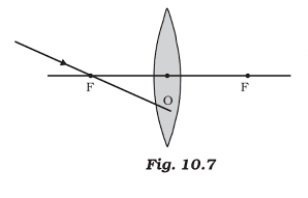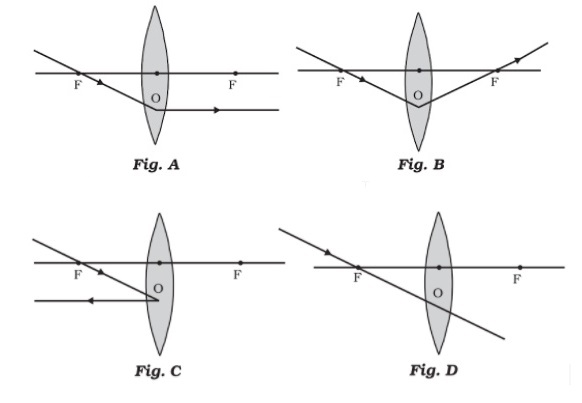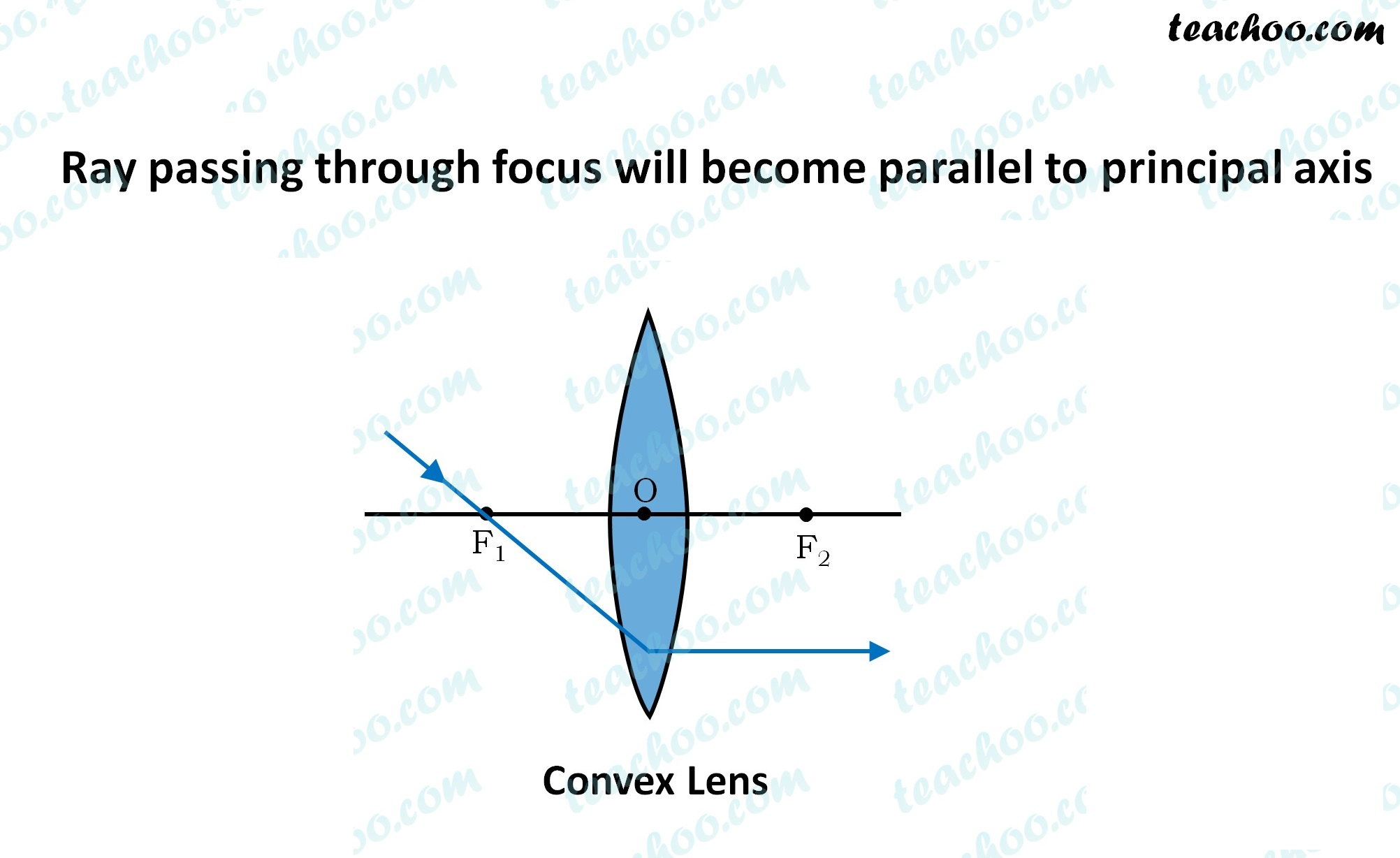MCQs from NCERT Exemplar

Class 10
Chapter 10 Class 10 - Light - Reflection and Refraction (Term 1)

## Which of the following ray diagrams is correct for the ray of light incident on a lens shown in Fig. 10.7?## (d) Fig. D.The figure given is that of a convex lens .

According to rules of drawing ray diagram

• For a lens, a ray of light passing through the focus of a convex lens
•   becomes parallel to the principal axis on the other side.So, the correct answer is (a)